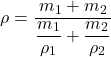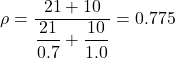## 4. Alcohol of mass 21g and density 0.7gcm-3 is mixed with 10g of water. Determine the density of the mixture. (density of water = 1.0g

Question

4. Alcohol of mass 21g and density 0.7gcm-3 is mixed with 10g of water. Determine the density
of the mixture. (density of water = 1.0g cm-3)

in progress 0
7 months 2021-07-16T10:09:15+00:00 1 Answers 10 views 0

The density of the mixture is 0.775 g/cm³

Explanation:

The given parameters are;

The mass of the alcohol, m₁ = 21 g

The density of the alcohol, ρ₁ = 0.7 g/cm³

The mass of the water with which the alcohol is mixed, m₂ = 10 g

The density of wate, ρ₂ = 1.0 g/cm³

The density of a substance is given by the ratio of the mass to the volume of the substance

The density of the mixture, ρ is given as follows;Therefore;The density of the mixture, ρ = 0.775 g/cm³.